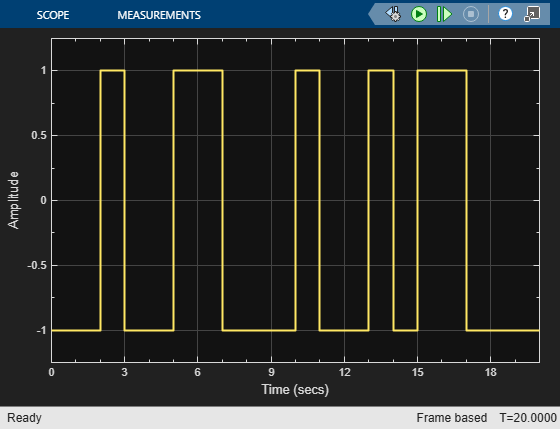# Bernoulli Binary Generator

Generate Bernoulli-distributed random binary numbers

•Libraries:
Communications Toolbox / Comm Sources / Random Data Sources

## Description

The Bernoulli Binary Generator block generates random binary numbers using a Bernoulli distribution. Use this block to generate random data bits to simulate digital communication systems and obtain performance metrics such as bit error rate. The Bernoulli distribution with parameter p produces zero with probability p and one with probability 1-p. The Bernoulli distribution has mean value 1-p and variance p(1-p). The Probability of zero parameter specifies p and can be any real number in range [0, 1].

The output signal can be a column or row vector, two-dimensional matrix, or scalar. The number of rows in the output signal corresponds to the number of samples in one frame and is set by the Samples per frame parameter. The number of columns in the output signal corresponds to the number of channels and is set by the number of elements in the Probability of zero parameter. For more details, see Sources and Sinks in Communications Toolbox™ User's Guide

## Examples

expand all

Use the Open model button to open the Bernoulli generator model. The model generates binary data, applies BPSK modulation, and displays the output.Run the model.## Ports

### Output

expand all

Output data signal, returned as a scalar, vector, or matrix.

Data Types: `double`

## Parameters

expand all

To edit block parameters interactively, use the Property Inspector. From the Simulink® Toolstrip, on the Simulation tab, in the Prepare gallery, select .

Probability of zero must be in the range of [0, 1]. The number of elements in the Probability of zero parameter corresponds to the number of independent channels output from the block. The Bernoulli distribution with parameter p produces zero with probability p and one with probability 1-p.

Select `Parameter` to use the Initial seed parameter to specify the initial seed for the random number generator.

Note

When the Source of initial seed parameter is set to `Auto` and the Simulate using parameter is set to `Code generation`, the random number generator uses an initial seed of zero. In this case, the block generates the same random numbers each time it is started. To ensure that the model uses different initial seeds, set Simulate using parameter to ```Interpreted execution```. If you run `Interpreted execution` in `Rapid accelerator` mode, then the model behaves the same as `Code generation` mode.

#### Dependencies

Select `Auto` for the block to use the global random number stream as the initial seed. For more information, see Managing the Global Stream Using RandStream and Random Number Generators.

If you set the Initial seed parameter to a constant value, then the resulting sequence is repeatable.

#### Dependencies

To enable this parameter, set the Source of initial seed to `Parameter`.

Positive scalars specify the time in seconds between each sample of the output signal. If you set the sample time to `-1`, the output signal inherits the sample time from downstream. For information on the relationship between the Sample time and Samples per frame parameters, see Sample Timing.

Samples per frame in one channel of the output signal, specified as a positive integer. For information on the relationship between Sample time and Samples per frame, see Sample Timing.

Select the data type for the output signal.

Type of simulation to run, specified as `Code generation` or `Interpreted execution`.

• `Code generation` — Simulate the model by using generated C code. The first time you run a simulation, Simulink generates C code for the block. The model reuses the C code for subsequent simulations unless the model changes. This option requires additional startup time, but the speed of the subsequent simulations is faster than with the `Interpreted execution` option.

• `Interpreted execution` — Simulate the model by using the MATLAB® interpreter. This option shortens startup time, but the speed of subsequent simulations is slower than with the ```Code generation``` option. In this mode, you can debug the source code of the block.

## Block Characteristics

 Data Types `Boolean` | `double` | `integer` | `single` Multidimensional Signals `no` Variable-Size Signals `no`

expand all

## Version History

Introduced before R2006a

expand all